Area of similar triangles

Chapter 6 Class 10 Triangles
Concept wise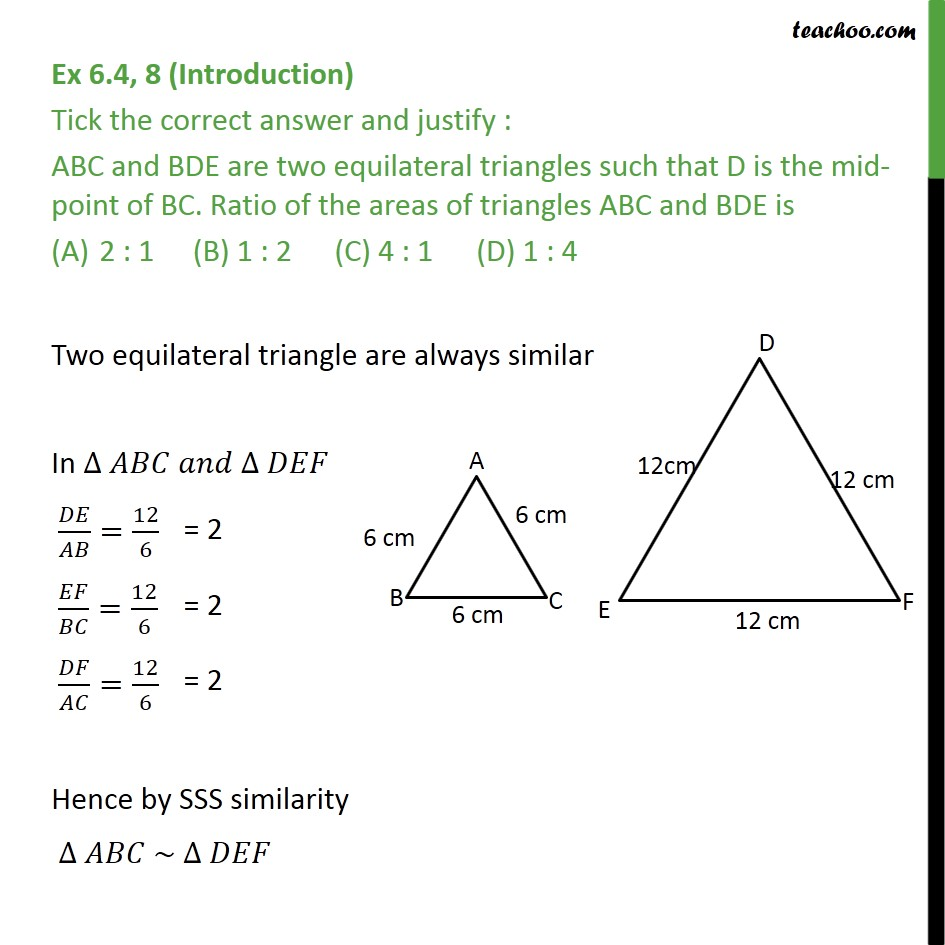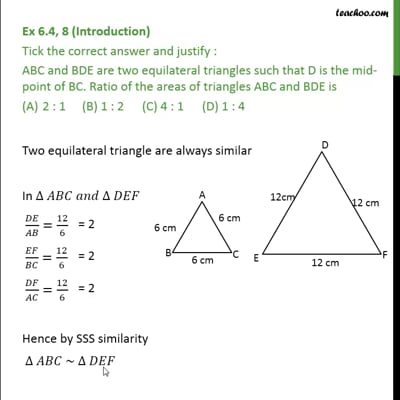This video is only available for Teachoo black users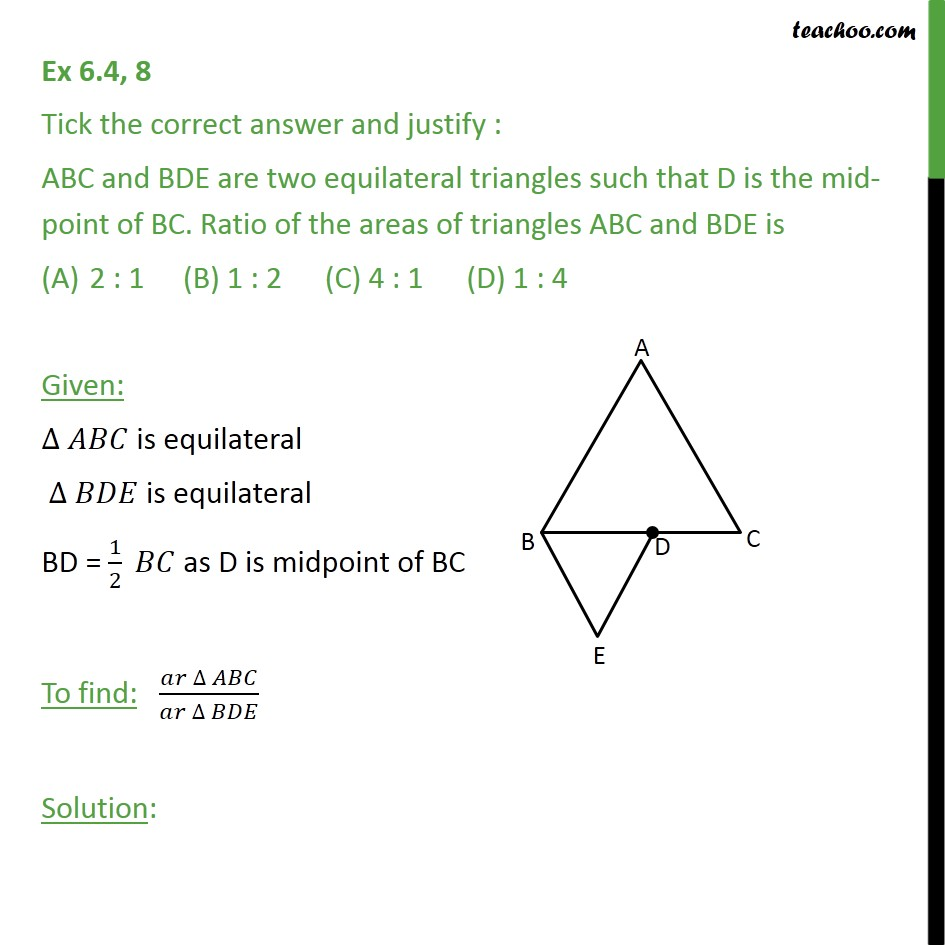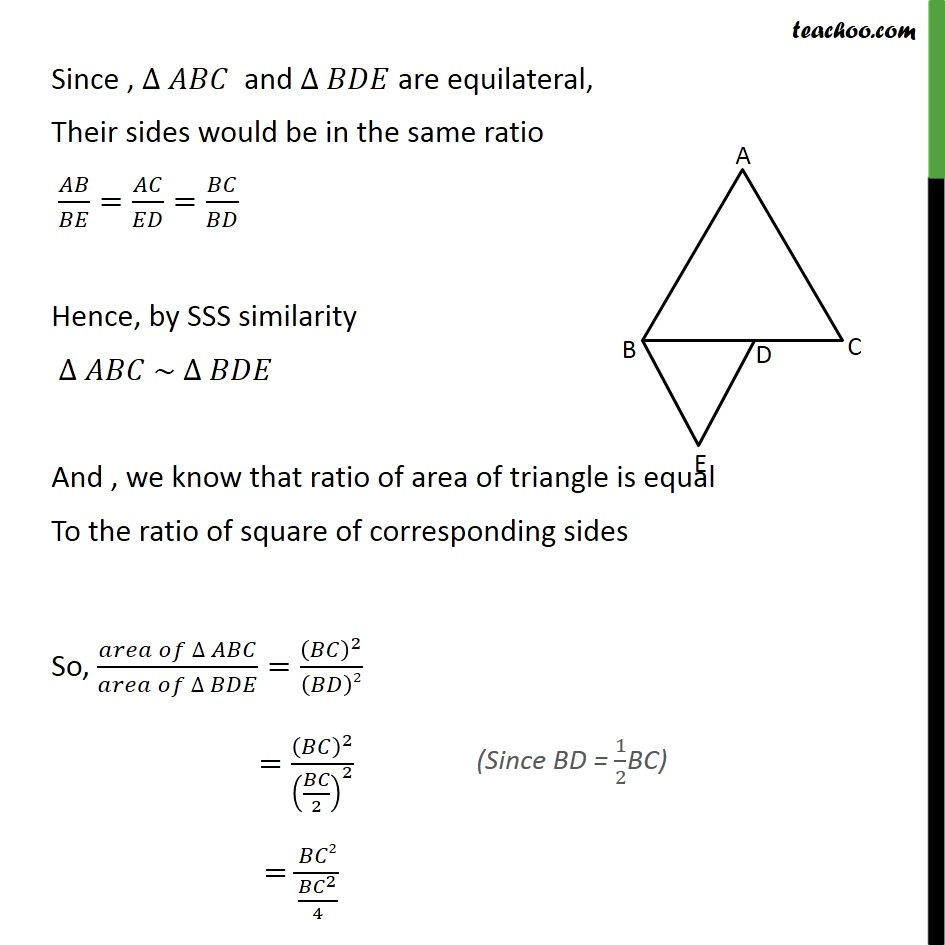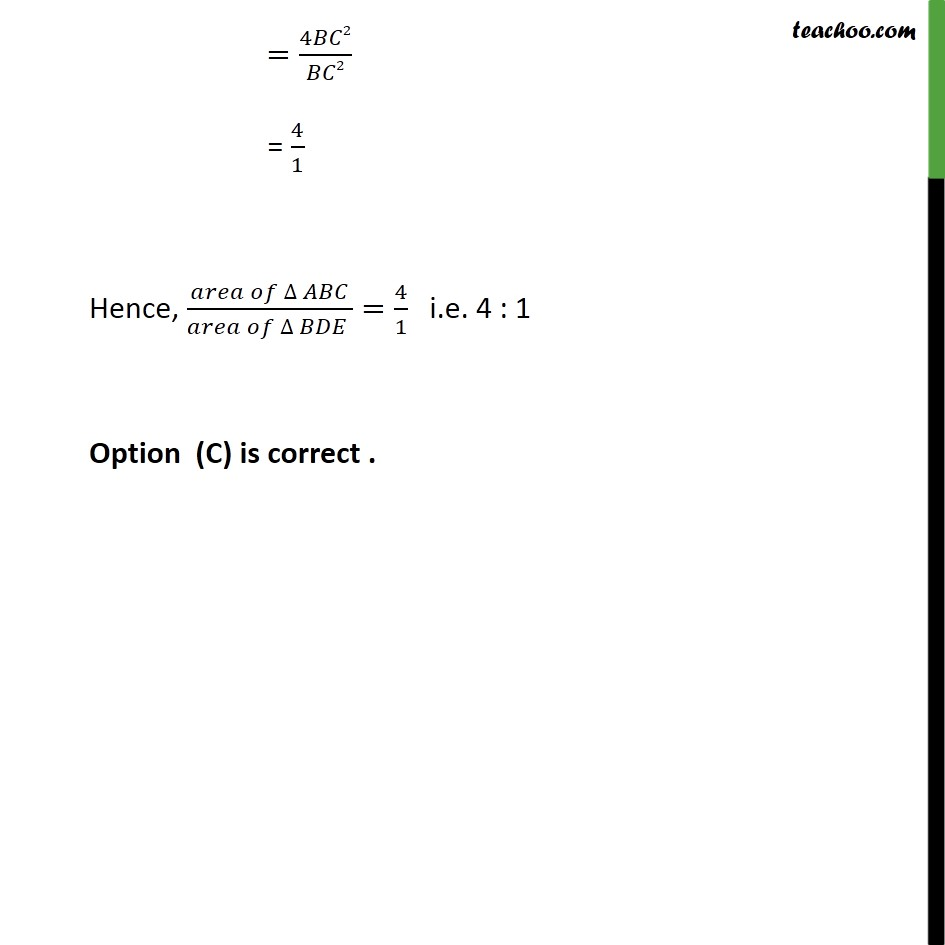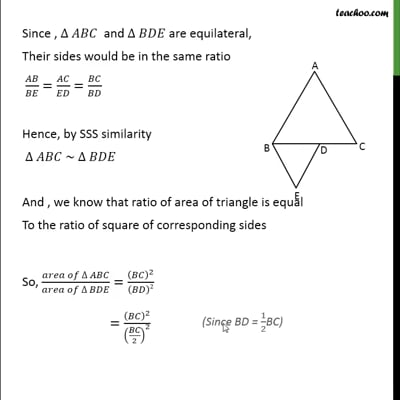This video is only available for Teachoo black users

Solve all your doubts with Teachoo Black (new monthly pack available now!)

### Transcript

Ex 6.4, 8 (Introduction) Tick the correct answer and justify : ABC and BDE are two equilateral triangles such that D is the mid-point of BC. Ratio of the areas of triangles ABC and BDE is 2 : 1 (B) 1 : 2 (C) 4 : 1 (D) 1 : 4 Two equilateral triangle are always similar In = 12 6 = 12 6 = 12 6 Hence by SSS similarity ~ Ex 6.4, 8 Tick the correct answer and justify : ABC and BDE are two equilateral triangles such that D is the mid-point of BC. Ratio of the areas of triangles ABC and BDE is 2 : 1 (B) 1 : 2 (C) 4 : 1 (D) 1 : 4 Given: is equilateral is equilateral BD = 1/2 as D is midpoint of BC To find: ( )/( ) Solution: Since , and are equilateral, Their sides would be in the same ratio / = / = / Hence, by SSS similarity ~ And , we know that ratio of area of triangle is equal To the ratio of square of corresponding sides So, ( )/( )=( )^2/( )2 =( )^2/( /2)^2 = 2/(( ^2)/4) =4 2/ 2 = 4/1 Hence, ( )/( )=4/1 i.e. 4 : 1 Option (C) is correct